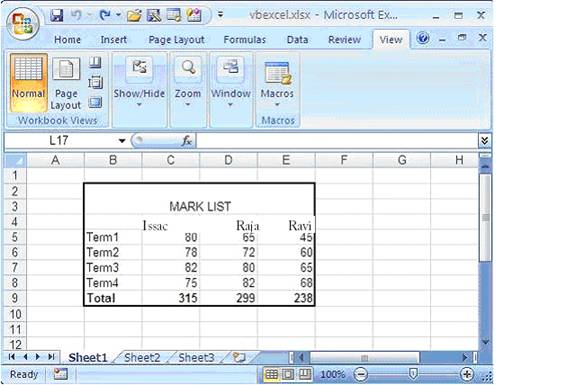Format Excel 2007 Page in VB.NET

 We can see some common formatting we can do in Excel 2007 page. For that let’s enter mark sheet data into a file and format it,  Excel sheet we gonna work will look something like this as we see in the following picture.``````Imports Excel = Microsoft.Office.Interop.Excel Public Class Form1 Private Sub Button1_Click(ByVal sender As System.Object, _ ByVal e As System.EventArgs) Handles Button1.Click Dim xlApp As Excel.Application Dim xlWorkBook As Excel.Workbook Dim xlWorkSheet As Excel.Worksheet Dim misValue As Object = System.Reflection.Missing.Value Dim chartRange As Excel.Range xlApp = New Excel.ApplicationClass xlWorkBook = xlApp.Workbooks.Add(misValue) xlWorkSheet = xlWorkBook.Sheets("sheet1") 'add data xlWorkSheet.Cells(4, 2) = "" xlWorkSheet.Cells(4, 3) = "Issac" xlWorkSheet.Cells(4, 4) = "Raja" xlWorkSheet.Cells(4, 5) = "Ravi" xlWorkSheet.Cells(5, 2) = "Term1" xlWorkSheet.Cells(5, 3) = "80" xlWorkSheet.Cells(5, 4) = "65" xlWorkSheet.Cells(5, 5) = "45" xlWorkSheet.Cells(6, 2) = "Term2" xlWorkSheet.Cells(6, 3) = "78" xlWorkSheet.Cells(6, 4) = "72" xlWorkSheet.Cells(6, 5) = "60" xlWorkSheet.Cells(7, 2) = "Term3" xlWorkSheet.Cells(7, 3) = "82" xlWorkSheet.Cells(7, 4) = "80" xlWorkSheet.Cells(7, 5) = "65" xlWorkSheet.Cells(8, 2) = "Term4" xlWorkSheet.Cells(8, 3) = "75" xlWorkSheet.Cells(8, 4) = "82" xlWorkSheet.Cells(8, 5) = "68" xlWorkSheet.Cells(9, 2) = "Total" xlWorkSheet.Cells(9, 3) = "315" xlWorkSheet.Cells(9, 4) = "299" xlWorkSheet.Cells(9, 5) = "238" chartRange = xlWorkSheet.Range("b2", "e3") chartRange.Merge() chartRange.FormulaR1C1 = "MARK LIST" chartRange.HorizontalAlignment = 3 chartRange.VerticalAlignment = 3 chartRange = xlWorkSheet.Range("b4", "e4") chartRange.Font.Bold = True chartRange = xlWorkSheet.Range("b9", "e9") chartRange.Font.Bold = True chartRange = xlWorkSheet.Range("b2", "e9") chartRange.BorderAround(Excel.XlLineStyle.xlContinuous, _ Excel.XlBorderWeight.xlMedium, Excel.XlColorIndex. _ xlColorIndexAutomatic,Excel.XlColorIndex.xlColorIndexAutomatic) xlWorkSheet.SaveAs("C:\vbexcel.xlsx") xlWorkBook.Close() xlApp.Quit() releaseObject(xlApp) releaseObject(xlWorkBook) releaseObject(xlWorkSheet) MsgBox("File created !") End Sub Private Sub releaseObject(ByVal obj As Object) Try System.Runtime.InteropServices.Marshal.ReleaseComObject(obj) obj = Nothing Catch ex As Exception obj = Nothing Finally GC.Collect() End Try End Sub End Class`````` When you run this code you will get a formated MarkList.